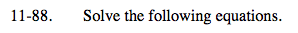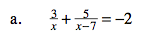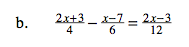### Home > A2C > Chapter Ch11 > Lesson 11.2.4 > Problem11-88

11-88.Multiply the entire equation by x and x − 7.

3x − 21 + 5x = −2x2 + 14x

Set the equation into standard format.

2x2 − 6x − 21 = 0

$x=\frac{6\pm\sqrt{36+168}}{4}$

$x=\frac{3\pm\sqrt{51}}{2}$Multiply by the entire equation by 12.

6x + 9 − 2x + 14 = 2x − 3

Solve for x.

2x = −26

x = −13